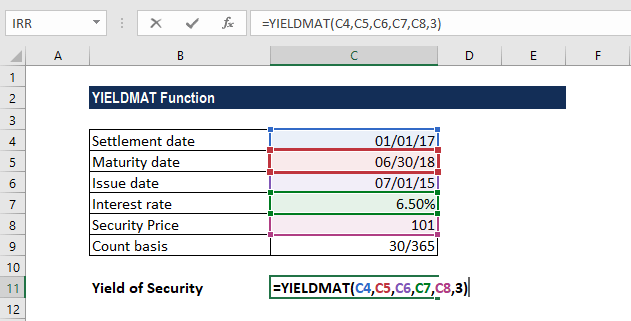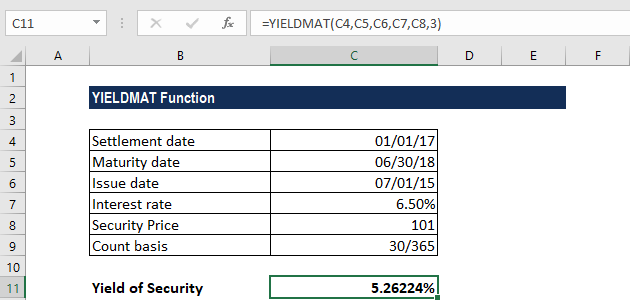# YIELDMAT Function

Returns the annual yield on a security that pays an interest at maturity

## What is the YIELDMAT Function?

The YIELDMAT function is categorized under Excel FINANCIAL functions. It will return the annual yield of a security that pays interest at maturity.

In financial analysis, YIELDMAT can be useful in calculating the yield on a bond that pays interest at maturity. The annual yield is the total increase in value in one year.

### Formula

=YIELDMAT(settlement, maturity, issue, rate, pr, [basis])

The YIELDMAT function uses the following arguments:

1. Settlement (required argument) – This is the settlement date of the security. The date should be after the issue date when the security is traded to the buyer.

The settlement date is the date a buyer purchases a security such as a bond. The maturity date is the date when the coupon expires. For example, suppose a 30-year bond is issued on January 1, 2009, and is purchased by a buyer six months later. The issue date would be January 1, 2009, the settlement date would be July 1, 2009, and the maturity date would be January 1, 2039, which is 30 years after the issue date of January 1, 2009.

1. Maturity (required argument) – The maturity date of the security or when the security expires.
2. Issue (required argument) – This is the issue date of the security. It is expressed as a serial date number.
3. Rate (required argument) – The interest rate of the security at the date of issue.
4. Pr (required argument) – This is the security’s price per \$100 face value.
5. Basis (optional argument) – The type of count day basis to use:

BasisDay Count basis
0 or omittedUS(NASD) 30/360
1Actual/actual
2Actual/360
3Actual/365
4European 30/360

### How to use the YIELDMAT Function in Excel?

As a worksheet function, YIELDMAT can be entered as part of a formula in a cell of a worksheet. To understand the uses of the function, let us consider an example:

#### Example

Let us calculate the annual yield for a security using the following details:

• Settlement date: January 1, 2017
• Maturity date: June 30, 2018
• Issue date: July 1, 2015
• Interest rate: 6.5%
• Security price: 101
• Count basis: Actual/365

The formula used here is:We get the results below:Note that in the above example:

• The yield of the security so calculated is formatted as a percentage.
• As recommended, the settlement, maturity, and issue dates were entered as references to cells that contain dates.

### A few notes about the YIELDMAT Function:

1. #NUM! error – Occurs if either:
1. The issue date is greater than or equal to the settlement date.
2. The settlement date is greater than or equal to the maturity date.
3. Invalid numbers are provided for the rate, pr or [basis] arguments, i.e., if either: rate < 0; pr ≤ 0; or [basis] is any number other than 0, 1, 2, 3, or 4).
2. #VALUE! error – Occurs if either:
1. Any of the given arguments are non-numeric.
2. The given settlement, maturity, or issue dates are not valid Excel dates.
3. MS Excel stores dates as sequential serial numbers so they can be used in calculations. By default, January 1, 1900 is serial number 1, and January 1, 2008 is serial number 39448 because it is 39,448 days after January 1, 1900.
4. Settlement, maturity, issue, and basis are truncated to integers.

Thanks for reading CFI’s guide to the Excel YIELDMAT function. By taking the time to learn and master these functions, you’ll significantly speed up your financial analysis. To learn more, check out these additional CFI resources:

• Excel Functions for Finance